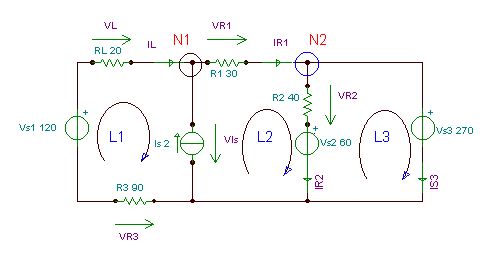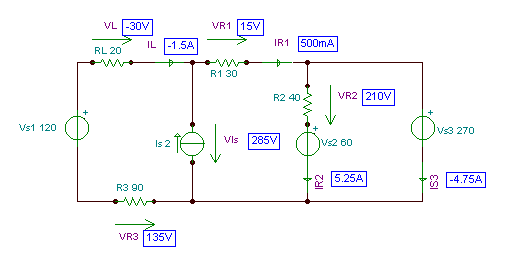# 基尔霍夫定律单击或点击下面的示例电路以调用TINACloud并选择交互式DC模式以在线分析它们。I = VS /（R1+R2+R3）= 120 /（10 + 20 + 30）= 2 A.

V1= I * R1 = 2 * 10 = 20 V; V2 = I * R.2 = 2 * 20 = 40 V; V3 = I * R.3 = 2 * 30 = 60 V.I1 I2 - 一世3 = 0

I1 + I2 +我3 = 0

-V1+I1*R1-I3*R3 = 0

I3*R3 - 一世2*R2 +V2 = 0

I1+我2+我3 = 0 -8 + 40 * I1 - 40 *我3 = 0 40 * I3 -20 * I2 + 16 = 0

-V1 - （一世2 +我3）* R1 -一世3*R3 = 0 or -8-（我2 +我3）* 40 - 我3* 40 = 0

I2 = –（V1 +我3*（R1+R3））/ R1 or I2 = - （8 + I3* 80）/ 40

I3*R3 + R.2*（V1 +我3*（R1+R3））/ R1 +V2 = 0 or I3* 40 + 20 *（8 + I3* 80）/ 40 + 16 = 0

 {TINA口译员的解决方案} 系统I1，I2，I3 I1 + I2 + I3 = 0 -V1+I1*R1-I3*R3=0 I3*R3-I2*R2+V2=0 结束; I1 = [ - 50m] I2 = [300m] I3 = [ - 250m]单击/点击上面的电路以在线分析，或单击此链接以在Windows下保存

-IL +我R1 - 一世s = 0 （对于N1）

- 一世R1 +我R2 +我s3 = 0 （对于N2）

-Vs1 - V.R3 + VIs + VL = 0 （对于L1）

-VIs + Vs2 +VR2 +VR1 = 0 （对于L2）

-VR2 - V.s2 + Vs3 = 0 （对于L3）

VL 我是L*RL

VR1 =IR1*R1

VR2 我是R2*R2

VR3 = –我L*R3

-IL +我R1 - 一世s = 0 （对于N1）

- 一世R1 +我R2 +我s3 = 0 （对于N2）

-Vs1 +我L*R3 + VIs +我L*RL = 0 （对于L1）

-VIs + Vs2 +我R2*R2 +我R1*R1 = 0 （用于 L2）

- 一世R2*R2 - V.s2 + Vs3 = 0 （对于L3）

-IL +我R1 - 一世s = 0 （对于N1）

- 一世R1 +我R2 +我s3 = 0 （对于N2）

-Vs1 +我L*R3 +我L*RL + Vs2 +我R2*R2 +我R1*R1 = 0 （L1）+（L2）

- 一世R2*R2 - V.s2 + Vs3 = 0 （对于L3）

-IL+IR1 - 2 = 0 （对于N1）

-IR1 +我R2 +我S3 = 0 （对于N2）

-120 – +我L* 90 +我L* 20 + 60 + I.R2* 40 +我R1* 30 = 0（L1）+（长2)

-IR2* 40 - 60 + 270 = 0 （对于L.3)

VR3 = –我L*R3= 135 V; VL 我是L*RL = – 30 V； VIs V =S1+VR3-VL = 285 V.

 {TINA的口译员对原始方程的求解} Sys IL,IR1,IR2,Is3,VIs,VL,VR1,VR3,VR2 -IL-IS + IR1 = 0 -IR1 + IR2 + Is3 = 0 -Vs1 + VR3 +显示-VL = 0 -Vis + VR1 + VR2 + Vs2 = 0 -Vs3 + VR2 + Vs2 = 0 VR1 = IR1 * R1 VR2 = IR2 * R2 VR3 = -IL * R3 VL = IL * RL 结束; IL = [ - 1.5] IR1 = [500m] IR2 = [5.25] Is3 = [ - 4.75] 的VI =  VL = [ - 30] VR1 =  VR2 =  VR3 = 

 {TINA的口译员对简化的方程组的求解} 系统II，Ir1，Ir2，Is3 -il + Ir1-2 = 0 -Ir1 + Ir2 + Is3 = 0 -120+110*Il+60+40*Ir2+30*Ir1=0 -40 * Ir2 + 210 = 0 结束; IL = [ - 1.5] Ir1 = [500m] Ir2 = [5.25] Is3 = [ - 4.75]

 IL：= - 1.5; Ir1：= 0.5; Ir2：= 5.25; Is3：= - 4.75; VL：= 11 * RL; Vr1：= Ir1 * R1 Vr2：= Ir2 * R2; Vr3：= - 金正日* R3; 的VI：= Vs1-VL + Vr3; VL = [ - 30] Vr1 =  Vr2 =  Vr3 =  的VI =X很高兴有你 DesignSoft
如果需要任何帮助找到合适的产品或需要支持，可以进行聊天。